### Home > MC1 > Chapter 3 > Lesson 3.1.2 > Problem3-20

3-20.

Copy and complete each of the Diamond Problems below. The pattern used in the Diamond Problems is shown at right.

1.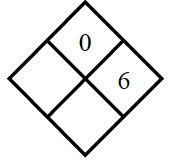You'll know you've found the right number when the product equals $0$.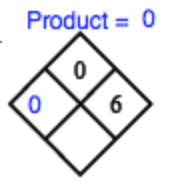Remember to find the sum once you have found the other number.

1.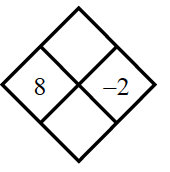Use the pattern to complete the diamonds in parts (b) and (c).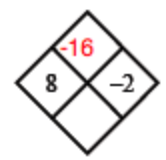1.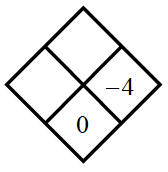Use the pattern to complete the diamonds in parts (b) and (c).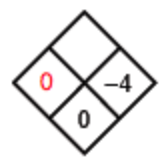1.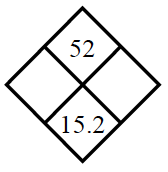Try using the number 10 as one of the side numbers. What happens?